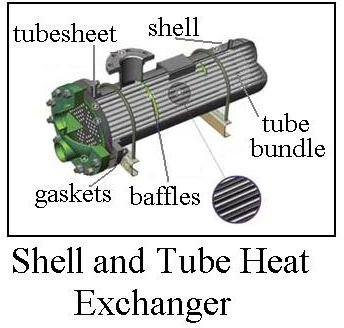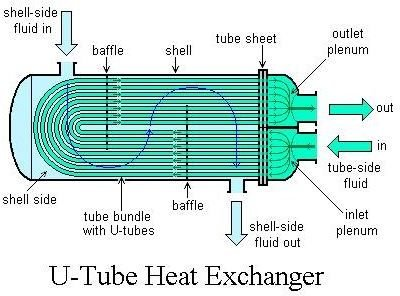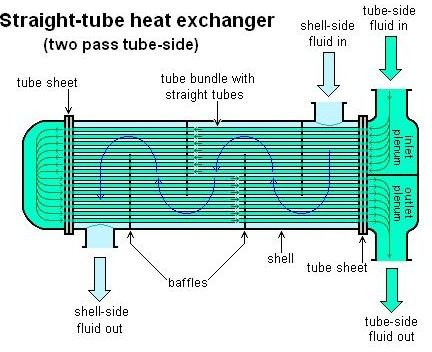# Shell and Tube Heat Exchanger: Design & Water Flow through it

## Introduction

The flow pattern in a shell and tube heat exchanger is typically not exactly counterflow or parallel flow, as with a double pipe heat exchanger. Rather, the flow pattern is typically some mix of counterflow, parallel flow and crossflow. The log mean temperature difference, which is used for shell and tube heat exchanger design, works for most of the varied flow patterns that occur in this type of heat exchanger. The general configuration and terminology for shell and tube heat exchangers is covered in ‘Types of Shell and Tube Heat Exchangers‘. This article will pick up from there.

## Shell and Tube Heat Exchanger Flow PatternsInstead of simply one pipe inside another, as for a double pipe heat exchanger, a shell and tube heatexchanger uses multiple tubes in a bundle inside of a ‘shell’. This gives a more compact heat exchanger for a given heat transfer area, but the flow patterns are somewhat more complicated for a shell and tube heat exchanger. The diagram at the left shows the general configuration. Some of the shell and tube heat exchanger options that affect the flow pattern are U-tube or straight tube and how many passes (tube side). The shell side flow pattern is determined by baffles as shown in the diagrams.A u-tube shell and tube heat exchanger has the tube side fluid entering and exiting at the same end of theheat exchanger, with the fluid making a ‘u-turn’ through tube bends at the other end, as shown in the upper diagram at the right. A u-tube shell and tube heat exchanger will automatically have two tube passes.

A straight tube shell and tube heat exchanger has a tube sheet and a plenum at both ends as shown in the lower two diagrams. The straight tube heat exchanger shown at the right has one tube pass and the one on the left has two tube passes.

## Shell and Tube Heat Exchanger Design

The required heat transfer surface area for a shell and tube heat exchanger design is typically found from the basic heat exchanger equation: Q = UA ΔTlm, where:

Q = rate of heat transfer between the two heat exchanger fluids, Btu/hr,

U = overall heat transfer coefficient, BTU/hr-ft2oF,

A = heat transfer surface area, ft2,

ΔTlm = log mean temperature difference in oF, calculated from the inlet and outlet temperatures of the two fluids.

The basic heat exchanger equation, and the above parameters, are discussed in ‘Fundamentals of Heat Exchanger Design‘. An example calculation with the basic heat transfer equation is given in ‘Preliminary Heat Exchanger Design Example‘.

If the required flow rate and temperature change of one of the fluids is known along with the flow rate and one temperature for the other fluid (or both temperatures for the other fluid), an estimate for the heat transfer coefficient, and the shell and tube heat exchanger flow pattern to be used, then the required heat transfer area can be calculated using the basic heat exchanger equation.

After the needed heat transfer surface area is calculated, an appropriate diameter, length and number for the tubes can be determined and the shell can be sized and designed. The tube sheets and baffles will also need to be designed.

## Image Credits

Shell and tube heat exchanger cutaway diagram – https://www.daviddarling.info/encyclopedia/H/AE_heat_exchanger.html

Shell and tube heat exchanger flow diagrams – https://en.wikipedia.org/wiki/Shell_and_tube_heat_exchanger

## About the Author

Dr. Harlan Bengtson is a registered professional engineer with 30 years of university teaching experience in engineering science and civil engineering. He holds a PhD in Chemical Engineering.